# GRADE 4 – SAMPEL PAPER FINAL FMO 2021

Berikut ini adalah soal beserta kunci jawaban Fermat Mathematic Olympiad (FMO) 2021 grade 4 (Sc: Edukultur Indonesia)

A. Warm-up (4 points per question / No points deducted for wrong answers)

1. Which figure should be filled into the cell with the question mark?A)B)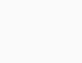C)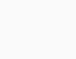D)E)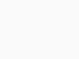2. Mr. Lee has a garden. He plants vegetable on $$\frac{1}{2}$$ of the garden; builds a pond on $$\frac{1}{3}$$ of the remaining land. Finally, he builds a path on the rest. Given that the area of the path is $$30m^2$$, find the area of the garden.
A) $$90m^2$$
B) $$60m^2$$
C) $$36m^2$$
D) $$80m^2$$
E) $$120m^2$$

3. Find the value of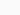given that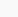is the smallest 3-digit odd number.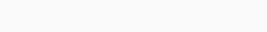A) 167
B) 170
C) 165
D) 169
E) 70

4. A pattern on a wall was created with 2 kinds of tiles: Grey and stripped so that tiles of the same type cannot be placed next to each other. Some tiles have fallen off the wall as in the picture to create a white region with perimeter 90cm. Find the total area (in $$cm^2$$) of all the grey tiles which have fallen off.A) 225
B) 200
C) 175
D) 150
E) 125

5. A store has 2 types of rice sacks. One weighs 50 kg and the other weighs 80kg. One day, they sell some rice sacks. Which answer below CANNOT be the total weight of rice sold in that day?
A) 100kg
B) 130kg
C) 210kg
D) 180kg
E) 170kg

B. Speed-up (6 points per question / No points deducted for wrong answers)

6. Alex folds the square paper in half twice and cuts it as the figure below.       How many pieces does he receive?A) 12
B) 10
C) 5
D) 4
E) 3

7. Five runners joined a race. The time each person took to finish the race is
shown in the graph with equally-spaced lines. After the winner crossed the
finishing line, he needs to wait 6 seconds for the third finisher. How many
seconds does it take for the last finisher to complete the race?A) 12
B) 18
C) 22
D) 33
E) 32

8. Altogether, there are 240 stamps owned by 4 collectors. Arthur gives Ben 3 books, Ben gives Charles 4 books, Charles gives Derek 5 books and Derek gives Arthur 6 books. Then each has the same number of stamps. Initially, how many stamps belong to the collector with the least number of stamps?
A) 57
B) 58
C) 59
D) 60
E) 61

9. Each cell has side length 1cm. Find the total area of 4 shapes below in $$cm^2$$.A) 44
B) 43
C) 41
D) 40
E) 42

10. .Teacher gives presents to each student one in 2 types of gift boxes: One with 2 pens and 3 books; the other with 5 pens and 1 book. Given that teacher has 17 pens and there are 7 students in the class. How many books does the teacher have?
A) 15
B) 19
C) 6
D) 21
E) 8

C. Challenge (8 points per question / No points deducted for wrong answers)

11. Candace glued 25 white blocks together and painted all the surface in red (except for the bottom). How many cube faces have been painted?A) 74
B) 79
C) 82
D) 70
E) 86

12. .A box contains 10 red, 5 green, 6 blue and 14 black balls. Ally wants to get 4 balls of different colors but she cannot look into the box. What is the minimum number of balls she should pick to be sure?
A) 29
B) 30
C) 31
D) 32
E) 33

13. .Eight identical rectangles with perimeter 36cm each are combined to form a big square as follows. Find the perimeter of the square.A) 84cm
B) 96cm
C) 144cm
D) 72cm
E) 108cm

14. Find the value of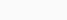A) 42
B) 66
C) 48
D) 57
E) None of the above

15. James wrote a different integer from 1 to 9 in each cell of $$3\times3$$ table. He calculated the sum of integers in each of the row and in each of the columns of the table. Five of his answers are 12, 13, 15, 16 and
17, in some order. What is his sixth answer?A) 17
B) 16
C) 15
D) 14
E) 13